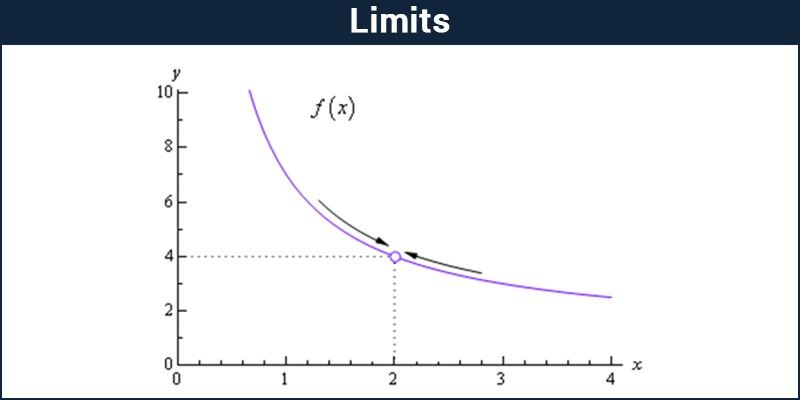Checkout JEE MAINS 2022 Question Paper Analysis : Checkout JEE MAINS 2022 Question Paper Analysis :

# Limits

In Mathematics, a limit is defined as a value that a function approaches the output for the given input values. Limits are important in calculus and mathematical analysis and used to define integrals, derivatives, and continuity. It is used in the analysis process, and it always concerns about the behaviour of the function at a particular point. The limit of a sequence is further generalized in the concept of the limit of a topological net and related to the limit and direct limit in theory category. Generally, the integrals are classified into two types namely, definite and indefinite integrals. For definite integrals, the upper limit and lower limits are defined properly. Whereas in indefinite the integrals are expressed without limits, and it will have an arbitrary constant while integrating the function. In this article, we are going to discuss the definition and representation of limits, with properties and examples in detail.## Limits Definition

Let us consider a real-valued function “f” and the real number “c”, the limit is normally defined as

$$\begin{array}{l}\lim _{x\to c}f(x)=L}\end{array}$$

It is read as “the limit of f of x, as x approaches c equals L”. The “lim” shows limit, and fact that function f(x) approaches the limit L as x approaches c is described by the right arrow.

## Properties of Limits

The following is the list of properties of limits.

We assume that

$$\begin{array}{l}\lim\limits_{x \to a}f(x)\end{array}$$
and
$$\begin{array}{l}\lim\limits_{x \to a} g(x)\end{array}$$
exist and c is a constant. Then,

1. $$\begin{array}{l}\lim\limits_{x \to a} \left [ c.f(x) \right ]= c \lim\limits_{x \to a}f(x)\end{array}$$

You can factor a constant that is multiplicative out of a limit.

1. $$\begin{array}{l}\lim\limits_{x \to a} \left [ f(x)\pm g(x) \right ]= \lim\limits_{x \to a}f(x) \pm \lim\limits_{x \to a}g(x)\end{array}$$

To consider the limit of a sum of difference, select the limits individually and put them back with the corresponding sign. This fact works regardless of number of functions we seperated by “+” or “-”.

1. $$\begin{array}{l}\lim\limits_{x \to a} \left [ f(x) .g(x) \right ]= \lim\limits_{x \to a}f(x) . \lim\limits_{x \to a}g(x)\end{array}$$

Consider the limits of products similar to the limits of sums or differences. Just select the limit of the pieces and put back, and this is not limited to only two functions.

1. $$\begin{array}{l}\large \lim\limits_{x \to a} \left [ \frac{f(x)}{g(x)} \right ] = \frac{\lim\limits_{x \to a}f(x)}{\lim\limits_{x \to a}g(x)}\end{array}$$
,

provided

$$\begin{array}{l}\lim\limits_{x \to a}g(x) \neq 0\end{array}$$

As you can see, we need to bother only if the limit of the denominator is zero when operating the quotient limit. If it were zero, it ends up with a division by zero error.

1.
$$\begin{array}{l}\lim\limits_{x \to a} c = c\end{array}$$

The limit of a constant is only a constant. You can easily understand it by plotting graph of the function f(x) = c.

1. $$\begin{array}{l}\lim\limits_{x \to a} xn = an\end{array}$$

### Limits Examples

Example 1:

To Compute

$$\begin{array}{l}\mathbf{\lim \limits_{x \to -4} (5x^{2} + 8x – 3)}\end{array}$$

Solution:

First, use property 2 to divide the limit into three separate limits. Then use property 1 to bring the constants out of the first two. This gives,

$$\begin{array}{l}\lim\limits_{x \to -4} (5x^{2}+8x-3) = \lim\limits_{x \to -4} (5x^{2})+ \lim\limits_{x \to -4} (8x)- \lim\limits_{x \to -4} (3)\end{array}$$

$$\begin{array}{l}=5(-4)^{2}+ 8(-4)- 3 \end{array}$$

$$\begin{array}{l}= 80 – 32 – 3 =45\end{array}$$

Example 2:

To Compute

$$\begin{array}{l}\mathbf{\lim\limits_{x \to 6} \left [ \frac{(x-3)(x-2)}{x-4} \right ]}\end{array}$$

Solution:

Given

$$\begin{array}{l}\lim\limits_{x \to 6} \left [ \frac{(x-3)(x-2)}{x-4} \right ]\end{array}$$

$$\begin{array}{l}= \left [ \frac{ \lim\limits_{x \to 6} (x-3) \lim\limits_{x \to 6}(x-2)}{ \lim\limits_{x \to 6}(x-4)} \right ]\end{array}$$

$$\begin{array}{l}= \left [ \frac{ (6-3) (6-2)}{ (6-4)} \right ]\end{array}$$

$$\begin{array}{l}= \left [ \frac{ (3) (4)}{ (2)} \right ] =6\end{array}$$

Example 3:

Compute

$$\begin{array}{l}\mathbf{\lim \limits_{x \to 3} \frac{(x^{2}- 9)}{x – 3}}\end{array}$$

Solution: Given

$$\begin{array}{l}\lim \limits_{x \to 3} \frac{(x^{2}- 9)}{x – 3}\end{array}$$

It is to be noted that, on substituting the value 3 directly to the funciton, the nemerator as well as denominator will become 0, and we know the value
$$\begin{array}{l}\frac{0}{0}\end{array}$$
, does not exist.

Using the property of squares , we have:

$$\begin{array}{l}\lim \limits_{x \to 3} \frac{(x-3)(x+3)}{x – 3} = \lim \limits_{x \to 3}(x+3)\end{array}$$

= 6

To solve more problems on limits of a function, Continuity and Differentiation, visit our site BYJU’S – The Learning App and explore more videos.